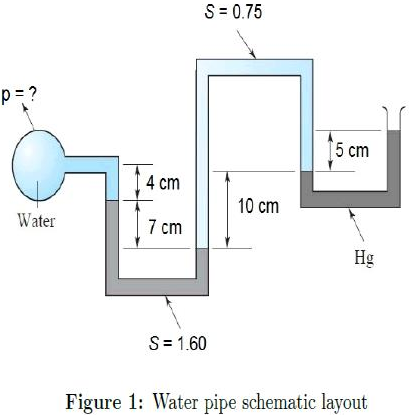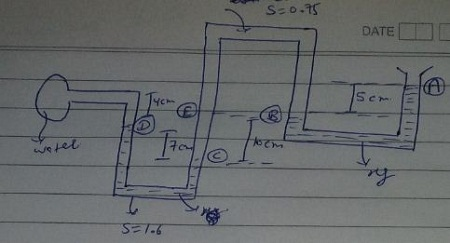# Find the pressure in the water tank shown in Figure 1

## Question:

Find the pressure in the water tank shown in Figure 1.## Calculating the pressure in a multilevel water tank:

Pressure is defined as force per unit area.

It is usually more convenient to use pressure rather than force to describe the influences upon fluid behavior.

The standard unit for pressure is the Pascal(Pa), which is a Newton/square meter.Now According to the above diagram,

{eq}P_A = 1atm = 101325 Pa {/eq}

= 1atm + 13600 * 9.81 * 0.05)Pa

= 101325 + 6670.8

=...

Become a Study.com member to unlock this answer! Create your account